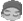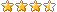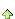共有6038人关注过本帖平板打印复制链接

# 主题：易表最新所得税公式1楼 | 信息 | 搜索 | 邮箱 | 主页 | UC加好友发短信易表最新所得税公式：if([实发工资] <= 5000,0,if(([实发工资]-5000) < 3000,([实发工资]-5000)*0.03,if(([实发工资]-5000) < 12000,([实发工资]-5000)*0.1-210,if(([实发工资]-5000) < 25000,([实发工资]-5000)*0.2-1410,if(([实发工资]-5000) < 35000,([实发工资]-5000)*0.25-2660,if(([实发工资]-5000) < 55000,([实发工资]-5000)*0.3-4410,if(([实发工资]-5000) < 80000,([实发工资]-5000)*0.35-7160,([实发工资]-5000)*0.45-15160)))))))